Do you like the article?
Share it with others -

Use new possibilities of MetaTrader 5

# Transferring an Indicator Code into an Expert Advisor Code. General Structural Schemes of an Expert Advisor and Indicator Functions

25 September 2007, 13:57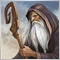1
8 723

### Introduction

In the previous article (Transferring an Indicator Code into an Expert Advisor Code. Indicator Structure) we analyzed the general structure of an indicator, the code of which is intended for transferring to an Expert Advisor code and described the main ideas of a preliminary adjustment of an indicator code. Now let us try to transform the obtained code into a custom function, because this is perhaps the most convenient way of presenting an indicator code in an Expert Advisor. A custom function may be presented as a mqh-file and its declaration in an Expert Advisor using the directive #include will take very little place, and calling this function is not much more difficult, than calling a custom indicator. What is more important, such custom functions can be rather universal for their further usage in any Expert Advisors.

Before we start writing such a function, let us analyze, how this function will interact with the other part of the Expert Advisor, irrespective of the function's inner structure.

### Structure of an EA with Custom Indicator Call

Let us first study the schematic structure of an EA that receives data from custom indicators. In this EA we are first of all interested only in the part that receives data from custom indicators. So far we will not discuss the way the EA processes these data, transferring them into trade signals and the structure of an executive part of the EA. Let us analyze as a custom indicator in this EA the one, discussed in the previous article. Here is an example of an Expert Advisor structure:

```//+------------------------------------------------------------------+
//|                                               ExpertIndPlan0.mqh |
//|                                       https://www.metaquotes.net/ |
//+------------------------------------------------------------------+
//---- EA input parameters
extern int period10 = 15;
extern int period11 = 15;
extern int period12 = 15;
//---- EA input parameters
extern int period20 = 15;
extern int period21 = 15;
extern int period22 = 15;
//---- Declaring buffers for indicator values
double Ind_Buffer1;
double Ind_Buffer2;
//+------------------------------------------------------------------+
//| Custom Expert initialization function                            |
//+------------------------------------------------------------------+
int init()
{
// Here is the code of EA initialization
//---- initialization end
return(0);
}
//+------------------------------------------------------------------+
//| Custom Expert iteration function                                 |
//+------------------------------------------------------------------+
int start()
{
//---- Checking whether the bars number is enough for further calculation
if(iBars(Symbol(), 0) < period10 + period11 + period12 + 10)
return(0);
if(iBars(Symbol(), 0) < period20 + period21 + period22 + 10)
return(0);
//---- getting indicator values for further calculation
for(int bar = 5; bar >= 1; bar--)
{
Ind_Buffer1[bar] = iCustom("IndicatorPlan", Symbol(), 0, period10,
period11, period12, 0, bar);
Ind_Buffer2[bar] = iCustom("IndicatorPlan", Symbol(), 0, period20,
period21, period22, 0, bar);
}
// Here is the EA code, forming trade signals
// based on the values of indicator buffer cells
//----
// here is the EA executive part code,
// requesting for placing an order
//----
return(0);
}
//+------------------------------------------------------------------+```

In this scheme on each tick we take from a zero buffer of the custom indicator IndicatorPlan. mq4 counted values in two calls and place them in common arrays Ind_Buffer1[] and Ind_Buffer2[]. The scheme of calling an indicator is made considering that for further calculations we will need only five last indicator values, except the zero one.

Structure of an EA with Custom Function Call

While we are developing a universal indicator function, suitable for any Expert Advisor, it should send the received values to indicator buffer analogues, which will store indicator values for all bars of the chart. Of course, we could develop an indicator function, calling which would be completely analogous to calling a custom indicator, but writing such a function would take too much time and its code will be quite lengthy.

We can do it easier. This function should receive as input parameters the parameters of a custom indicator and buffer, and it should return the same buffer with an indicator mode emulation, where cells are filled with calculated indicator values. It can be easily done by declaring in our function an linked-by-reference external variable for function corresponding to an indicator buffer. In MQL4 language it will look like this: double& InputBuffer. The indicator function should be declared as a logical one, returning 'true', if a calculation was successful, or 'false', if a calculation was unsuccessful due to the absence of a proper number of bars on the chart. After these explanations the indicator function, built from the indicator scheme, discussed in the previous article, is supposed to have the following form:

```bool Get_IndSeries(int Number,string symbol, int timeframe,
bool NullBarRecount, int period0, int period1,
int period2, double& InputBuffer0[],
double& InputBuffer1[], double& InputBuffer2[])```

The indicator function has one more additional external variable Number, which accepts the value of this indicator function call number.

It is natural that except the indicator buffer InputBuffer0 the external variables will also contain buffers for intermediary calculations InputBuffer1 and InputBuffer2, because it is quite problematic to make these buffers inside the function. It is better to emulate the indicator mode of these buffers operation inside the function. It will not cause any problem. Now let us dwell on the meaning of the external variable NullBarRecount. Actually, the majority of EAs do not need calculations on the zero bar, and while we are writing a code of the universal indicator function, it will naturally recalculate indicator values on the zero bar, which may substantially increase the execution time. By indicating the external parameter of the function NullBarRecount as 'false', we prohibit the function calculations on the zero bar, if it is not necessary.

Now we can present the scheme of an EA structure for calling indicators in a variant with calling functions:

```//+------------------------------------------------------------------+
//|                                               ExpertIndPlan1.mqh |
//|                                       https://www.metaquotes.net/ |
//+------------------------------------------------------------------+
//---- EA input parameters
extern int period10 = 15;
extern int period11 = 15;
extern int period12 = 15;
//---- EA input parameters
extern int period20 = 15;
extern int period21 = 15;
extern int period22 = 15;
//---- Indicator buffers declaration
double Ind_Buffer10[], Ind_Buffer11[], Ind_Buffer12[];
double Ind_Buffer20[], Ind_Buffer21[], Ind_Buffer22[];
//+------------------------------------------------------------------+
//| Get_IndSeries() function                                         |
//+------------------------------------------------------------------+
//---- Declaration of the function Get_IndSeries()
bool Get_IndSeries(int Number,string symbol, int timeframe,
bool NullBarRecount, int period0, int period1,
int period2, double& InputBuffer0[],
double& InputBuffer1[], double& InputBuffer2[])
{
//----
// Here is the code of the function GetIdicator()
//----
return(true);
}
//+------------------------------------------------------------------+
//| Custom Expert initialization function                            |
//+------------------------------------------------------------------+
int init()
{
//----
// Here is the code of the EA initialization
//---- initialization end
return(0);
}
//+------------------------------------------------------------------+
//| Custom Expert iteration function                                 |
//+------------------------------------------------------------------+
int start()
{
//---- Checking whether the bars number is enough for further calculation
if(iBars(Symbol(), 0) < period10 + period11 + period12 + 10)
return(0);
if(iBars(Symbol(), 0) < period20 + period21 + period22 + 10)
return(0);
//---- getting indicator values for further calculation
if(!Get_IndSeries(0,Symbol(), 0, false, period10, period11, period12,
Ind_Buffer10, Ind_Buffer11, Ind_Buffer12))
return(0);
if(!Get_IndSeries(1, Symbol(), 0, false, period20, period21, period22,
Ind_Buffer20, Ind_Buffer21,Ind_Buffer22))
return(0);
//----
// Here is the EA code, forming trade signals
// based on the values of indicator buffer cells
//----
// here is the EA executive part code,
// requesting for placing an order
//----

return(0);
}
//+------------------------------------------------------------------+```

General Scheme of Transforming an Indicator Code into a Custom Function

After this preliminary work we can move to building a general scheme of an inner structure of an indicator function. Let us take as a basis the last indicator scheme of the previous article. There should not be any difficulties:

1. Take only the contents of the function int start();

2. Add declaration of the function Get_IndSeries():

```bool Get_IndSeries(string symbol, int timeframe, bool NullBarRecount,
int period0, int period1, int period2,
double& InputBuffer0, double& InputBuffer1,
double& InputBuffer2)```

3. Change the names of indicator buffers inside the code (Ind_Buffer) accordingly into buffer names (InputBuffer) of the external variables of the function Get_IndSeries();

4. Add declaration of the variable LastCountBar;

5. Check the truth of the variable NullBarRecount:

```if(!NullBarRecount)
LastCountBar = 1;```

6. In all cycles of the indicator calculation change zero into LastCountBar;

7. Make changes at the very beginning of the code, when checking whether the bars number is enough for further calculation: return(0) into return(false);

8. In the end of the code change return(0) into return(true);

```//+------------------------------------------------------------------+
//|                                                Get_IndSeries.mqh |
//|                                        https://www.metaquotes.net/ |
//+------------------------------------------------------------------+
bool Get_IndSeries(int Number, string symbol,int timeframe,
bool NullBarRecount, int period0, int period1,
int period2, double& InputBuffer0[],
double& InputBuffer1[], double& InputBuffer2[])
{
//---- getting the number of all bars of a chart
int IBARS = iBars(symbol, timeframe);
//---- Checking whether the bars number is enough for further calculation
if(IBARS < period0 + period1 + period2)
return(false);
//---- EMULATION OF INDICATOR BUFFERS
if(ArraySize(InputBuffer0) < IBARS)
{
ArraySetAsSeries(InputBuffer0, false);
ArraySetAsSeries(InputBuffer1, false);
ArraySetAsSeries(InputBuffer2, false);
//----
ArrayResize(InputBuffer0, IBARS);
ArrayResize(InputBuffer1, IBARS);
ArrayResize(InputBuffer2, IBARS);
//----
ArraySetAsSeries(InputBuffer0, true);
ArraySetAsSeries(InputBuffer1, true);
ArraySetAsSeries(InputBuffer2, true);
}
//----+ introducing static memory variables
static int IndCounted[];
//----+ changing the size of static variables
if(ArraySize(IndCounted) < Number + 1)
ArrayResize(IndCounted, Number + 1);
//----+ introducing an integer variable
int LastCountBar;
//----+ Checking if the recalculation of the zero bar is allowed
if(!NullBarRecount)
LastCountBar = 1;
else
LastCountBar = 0;
//----+ Inserting a variable with a floating point
double Resalt0, Resalt1, Resalt2;
//----+ Inserting integer variables and getting already calculated bars
int limit, MaxBar, bar, counted_bars = IndCounted[Number];
//----+ Remembering the number of all bars of a chart (we do not count the zero bar!)
IndCounted[Number] = IBARS - 1;
//---- defining the number of the oldest bar,
// starting from which new bars will be recalculated
limit = IBARS - counted_bars - 1;
//---- defining the number of the oldest bar,
// starting from which new bars will be recalculated
MaxBar = IBARS - 1 - (period0 + period1 + period2);
//---- initialization of zero
if(limit > MaxBar)
{
limit = MaxBar;
for(bar = IBARS - 1; bar >= 0; bar--)
{
InputBuffer0[bar] = 0.0;
InputBuffer1[bar] = 0.0;
InputBuffer2[bar] = 0.0;
}
}
//----+ THE FIRST CYCLE OF INDICATOR CALCULATION
for(bar = limit; bar >= LastCountBar; bar--)
{
// Here code of the variable Resalt1 calculation
// based on the external variable period1
InputBuffer1[bar] = Resalt1;
}
//----+ THE SECOND CYCLE OF INDICATOR CALCULATION
for(bar = limit; bar >= LastCountBar; bar--)
{
// Here code of the variable Resalt2 calculation
// based on the values of the buffer Ind_Buffer1[]
// and external variable period2
InputBuffer2[bar] = Resalt2;
}
//----+ THE MAIN CYCLE OF INDICATOR CALCULATION
for(bar = limit; bar >= LastCountBar; bar--)
{
// Here code of the variable Resalt0 calculation
// based on the values of the buffer Ind_Buffer2[]
// and external variable period0
InputBuffer0[bar] = Resalt0;
}
return(true);
}
//+------------------------------------------------------------------+```

I think if a reader uses MQL4 quite well, after reading the actions described above he will not have any problems in writing indicator functions according to the given scheme.

Example of Writing a Custom Indicator Function

Now let us write an indicator function. Let us take a maximally simple indicator:

```//+------------------------------------------------------------------+
//|                                                         RAVI.mq4 |
//|                                       https://www.metaquotes.net/ |
//+------------------------------------------------------------------+
//---- drawing the indicator in a separate window
#property indicator_separate_window
//---- number of indicator buffers
#property indicator_buffers 1
//---- indicator color
#property indicator_color1 Red
//---- INPUT PARAMETERS OF THE INDICATOR
extern int Period1 = 7;
extern int Period2 = 65;
extern int MA_Metod = 0;
extern int PRICE = 0;
//---- indicator buffers
double ExtBuffer[];
//+------------------------------------------------------------------+
//| RAVI initialization function                                     |
//+------------------------------------------------------------------+
int init()
{
//---- indicator drawing style
SetIndexStyle(0, DRAW_LINE);
//---- indicator buffers
SetIndexBuffer(0,ExtBuffer);
//---- indicator name and labels for subwindows
IndicatorShortName("RAVI (" + Period1+ ", " + Period2 + ")");
SetIndexLabel(0, "RAVI");
//---- initialization end
return(0);
}
//+------------------------------------------------------------------+
//| RAVI iteration function                                          |
//+------------------------------------------------------------------+
int start()
{
int MinBars = MathMax(Period1, Period2);
//---- checking whether the bars number is enough for further calculation
if(Bars < MinBars)
return(0);
//----+ Introducing variables with a floating point
double MA1, MA2, result;
//----+ Introducing integer variables and getting already calculated bars
int MaxBar, bar, limit, counted_bars = IndicatorCounted();
//---- checking for possible errors
if(counted_bars < 0)
return(-1);
//---- the last calculated bar should be recalculated
if(counted_bars > 0)
counted_bars--;
//---- defining the number of the oldest bar,
// starting from which all bars will be recalculated
MaxBar = Bars - 1 - MinBars;
//---- defining the number of the oldest bar,
// starting from which new bars will be recalculated
limit = Bars - counted_bars - 1;
//---- zero initialization
if(limit > MaxBar)
{
for(int ii = Bars - 1; ii >= MaxBar; ii--)
ExtBuffer[ii] = 0.0;
limit = MaxBar;
}
//---- main cycle
for(bar = 0; bar <= limit; bar++)
{
MA1 = iMA(NULL, 0, Period1, 0, MA_Metod, PRICE,bar);
MA2 = iMA(NULL, 0, Period2, 0, MA_Metod, PRICE,bar);
//----
result = ((MA1 - MA2) / MA2)*100;
ExtBuffer[bar] = result;
}
//----
return(0);
}
//+------------------------------------------------------------------+```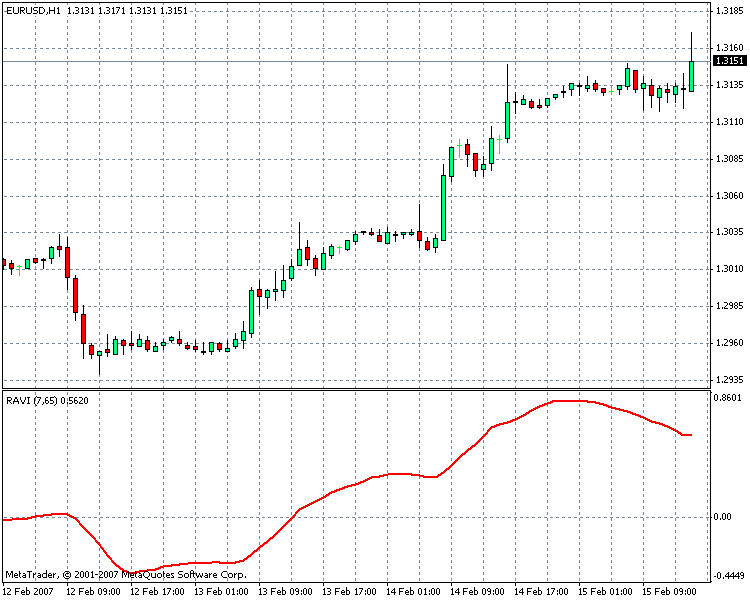Fixing Algorithm

1. Get rid of all unnecessary elements in the indicator code;

2. Write the code of indicator buffer emulation for a single buffer ExtBuffer[];

3. Substitute the function IndicatorCounted() for the variable IndCounted;

4. Initialize the variable IndCounted by the amount of the chart bars minus one;

5. Change the predetermined variable Bars into calling the timeseries iBars(symbol, timeframe);

6. Delete unnecessary checking for counted_bars:

```//---- checking possible errors
if(counted_bars < 0)
return(-1);
//---- the last calculated bar must be recalculated
if(counted_bars > 0)
counted_bars--;```

7. Leave only the contents of the function int start();

8. Add declaration of the function Get_RAVISeries():

```bool Get_RAVISeries(int Number, string symbol,int timeframe,
bool NullBarRecount, int Period1,
int Period2, int MA_Metod, int  PRICE,
double& InputBuffer[])```

9. Substitute indicator buffer names inside the code (ExtBuffer) accordingly for buffer names (InputBuffer) of external variables of the function Get_RAVISeries();

10. Add declaration of the variable LastCountBar;

11. Turn the static variable IndCounted into an array IndCounted[Number] and add a code for changing the size of variables depending on the number of calls of the function Get_RAVISeries. mqh:

```//----+ changing the size of static variables
if(ArraySize(IndCounted) < Number + 1)
{
ArrayResize(IndCounted, Number + 1);
}```

12. Check the truth of the variable NullBarRecount:

```if(!NullBarRecount)
LastCountBar = 1;```

13. In all indicator calculation cycles change zero into LastCountBar:

`for(bar = limit; bar >= LastCountBar; bar--)`

14. Make a change in the beginning of the code, when checking whether the bars number is enough: return(0) into return(false);

15. At the end substitute return(0) for return(true).

After all code changes we get the indicator function Get_RAVISeries():

```//+------------------------------------------------------------------+
//|                                               Get_RAVISeries.mqh |
//|                                       https://www.metaquotes.net/ |
//+------------------------------------------------------------------+
bool Get_RAVISeries(int Number, string symbol,int timeframe,
bool NullBarRecount, int Period1, int Period2,
int MA_Metod, int  PRICE, double& InputBuffer[])
{
//---- getting the number of all bars of a chart
int IBARS = iBars(symbol, timeframe);
//---- Checking whether the bars number is enough for further calculation
if(IBARS < MathMax(Period1, Period2))
return(false);
//---- EMULATION OF INDICATOR BUFFERS
if(ArraySize(InputBuffer) < IBARS)
{
ArraySetAsSeries(InputBuffer, false);
//----
ArrayResize(InputBuffer, IBARS);
//----
ArraySetAsSeries(InputBuffer, true);
}
//----+  inserting static variables of memory
static int IndCounted[];
//----+ changing the size of static variables
if(ArraySize(IndCounted) < Number + 1)
{
ArrayResize(IndCounted, Number + 1);
}
//----+ Introducing an integer variable
int LastCountBar;
//----+ Checking whether the recalculation of the zero bar is allowed
if(!NullBarRecount)
LastCountBar = 1;
//----+ Introducing floating point variables
double MA1,MA2,result;
//----+ Introducing integer variables and getting alreadu calculated bars
int MaxBar, bar, limit, counted_bars = IndCounted[Number];
//----+ Remembering the amount of all chart bars
IndCounted[Number] = IBARS - 1;
//---- determining the number of the oldest bar,
// starting from which new bars will be recalculated
limit = IBARS - counted_bars - 1;
// Print(IBARS - counted_bars);
//---- determining the number of the oldest bar,
// starting from which all bars will be recalculated
MaxBar = IBARS - 1 - MathMax(Period1, Period2);
//---- zero initialization
if(limit > MaxBar)
{
limit = MaxBar;
for(bar = IBARS - 1; bar >= 0; bar--)
{
InputBuffer[bar] = 0.0;
}
}
//----+ THE FIRST CYCLE OF INDICATOR CALCULATION
for(bar = limit; bar >= LastCountBar; bar--)
{
MA1 = iMA(symbol, timeframe, Period1, 0, MA_Metod, PRICE, bar);
MA2 = iMA(symbol, timeframe, Period2, 0, MA_Metod, PRICE, bar);
//----
result = ((MA1 - MA2) / MA2)*100;
InputBuffer[bar] = result;
}
//----+
return(true);
}
//+------------------------------------------------------------------+```

Certainly, all this is great! Competently, not too simple. But a question arises - will this indicator function calculate the same, as a custom indicator?

Testing the Custom Indicator Function for the Calculation Accuracy

We need to check, whether the function calculation results are equal to the results of a custom indicator's calculation. For this purpose the best suiting Expert Advisor is the one that does not trade and only receives values from the custom indicator RAVI.mq4 and the custom function Get_RAVISeries(), finds the difference and after that sends into a log-file the indicator value, the custom function value and the difference between them. All we need to do is analyze the contents of the log-file for making the final conclusion about the correspondence of our algorithm of the custom function Get_RAVISeries() and the indicator RAVI.mq4:

```//+------------------------------------------------------------------+
//|                                           Get_RAVISeriesTest.mq4 |
//|                                       https://www.metaquotes.net/ |
//+------------------------------------------------------------------+
//---- INPUT EA PARAMETERS
extern bool NullBarRecount = true;
//---- indicator buffers
double RAVI_Buffer0[];
double RAVI_Buffer1[];
double RAVI_Buffer2[];
//+------------------------------------------------------------------+
//| Get_RAVISeries() function                                        |
//+------------------------------------------------------------------+
#include <Get_RAVISeries.mqh>
//+------------------------------------------------------------------+
//| Custom Expert initialization function                            |
//+------------------------------------------------------------------+
int init()
{
//---- initialization end
return(0);
}
//+------------------------------------------------------------------+
//| Custom Expert iteration function                                 |
//+------------------------------------------------------------------+
int start()
{
//----
double Ind_Velue, Resalt;
//----
if(!Get_RAVISeries(0, Symbol(), 0, NullBarRecount, 10, 20, 1, 0,
RAVI_Buffer0))
return(0);
if(!Get_RAVISeries(1, Symbol(), 240, NullBarRecount, 25, 66, 2, 1,
RAVI_Buffer1))
return(0);
if(!Get_RAVISeries(2, Symbol(), 1440, NullBarRecount, 30, 70, 3, 3,
RAVI_Buffer2))
return(0);
//---- getting indicator values for the test 0
Ind_Velue = iCustom(NULL, 0, "RAVI", 10, 20, 1, 0, 0, 2);
Resalt = RAVI_Buffer0 - Ind_Velue;
Print("  " + Ind_Velue + "    " + RAVI_Buffer0 + "    " + Resalt+"");
//---- getting indicator values for the test 1
Ind_Velue = iCustom(NULL, 240, "RAVI", 25, 66, 2, 1, 0, 2);
Resalt = RAVI_Buffer1 - Ind_Velue;
Print("  " + Ind_Velue + "    " + RAVI_Buffer1 + "    " + Resalt+"" );
//---- getting indicator values for the test 2
Ind_Velue = iCustom(NULL, 1440, "RAVI", 30, 70, 3, 3, 0, 2);
Resalt = RAVI_Buffer2 - Ind_Velue;
Print("  " + Ind_Velue + "    " + RAVI_Buffer2 + "    " + Resalt + "");
//----
return(0);
}
//+------------------------------------------------------------------+```

In a strategy tester start the Expert Advisor Get_RAVISeriesTest. Naturally the compiled file RAVI.ex4 must already be in the folder \expert\indicators, and the file Get_RAVISeries.mqh in the folder \expert \include of MetaTrader Client Terminal. In strategy tester journal and in a log-file we see two columns with indicator values and its analogue in the form of a function; the third column shows the difference of these values. All values of the last column are equal to zero. It means the values are identical in both cases. We can conclude that the task of writing an indicator custom function is successfully solved!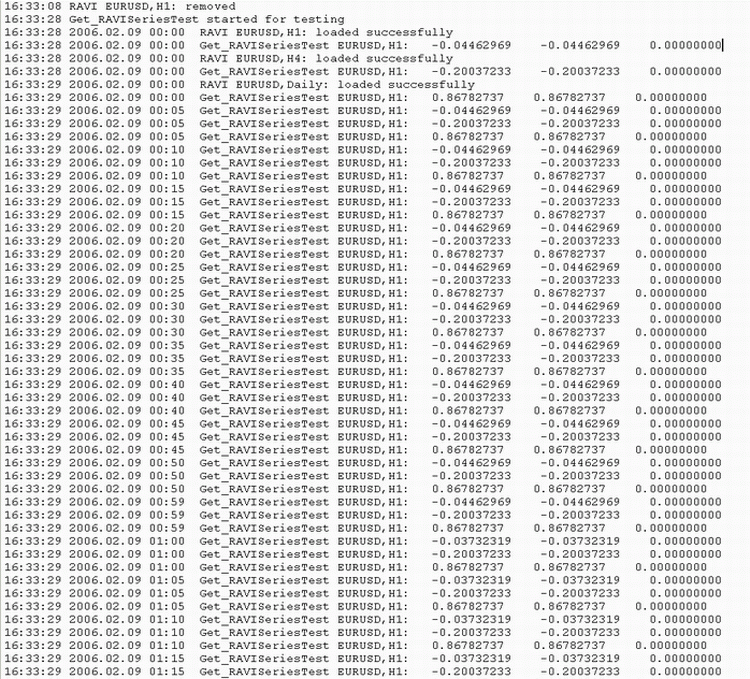### Conclusion

So we managed to solve the task of transferring an indicator code from a custom indicator to an Expert Advisor code by making an analogue of the indicator as a universal custom function, which may be placed in a mqh-file and used in the code of any Expert Advisor in the way analogous to a custom indicator.

In the next article, devoted to this topic, we will analyze a more difficult example of writing functions of this kind and implementing a simple Expert Advisor based on such functions.

Translated from Russian by MetaQuotes Software Corp.
Original article: https://www.mql5.com/ru/articles/1457

Attached files |
ExpertIndPlan0.mqh (2.27 KB)
ExpertIndPlan1.mqh (2.89 KB)
RAVI.mq4 (2.93 KB)
Last comments | Go to discussion (1)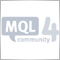| 13 Feb 2008 at 09:30

Hi Nikolay,

Thank you for your article. Excellent work!!

I used RAVI but I still get the old problem of a difference in values when I use the EA to call the CI and the values of the CI on the graph itself.

For Example, the value of RAVI CI attached to the graph is -0.3127 on 2006.02.10 12:00.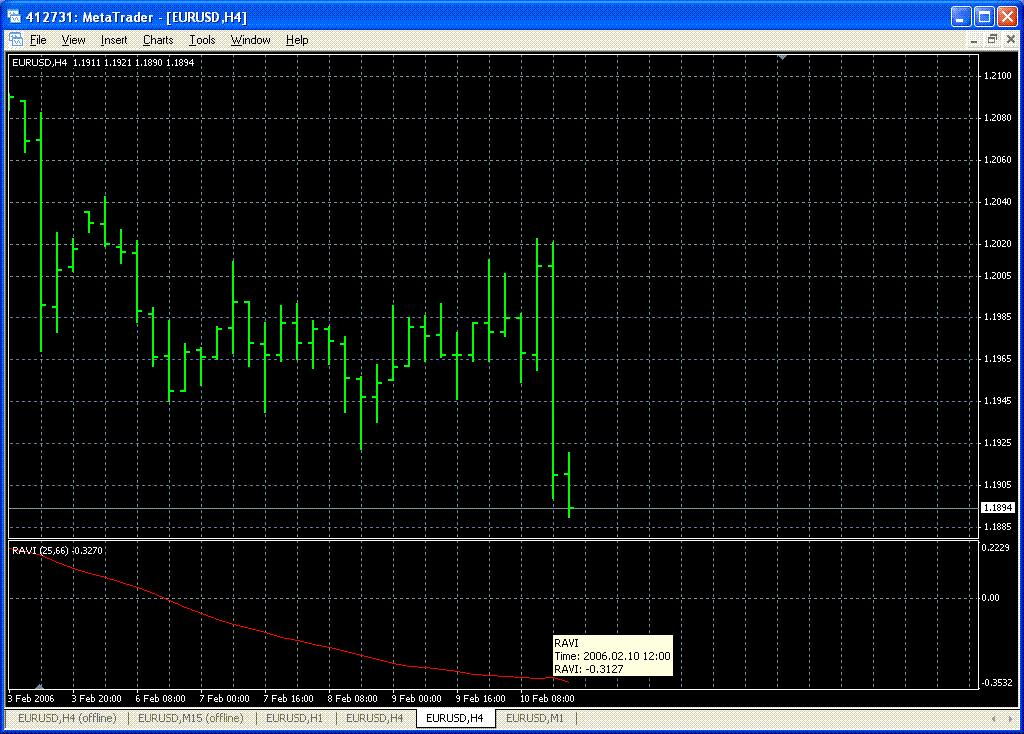The value of  Get_RaviSeriesTest is -0.30348183 for the same date and the same timeframe.

This the output on Tick basesed backtesting:

07:52:25 2006.02.10 12:00  Get_RAVISeriesTest EURUSD,H4:   -0.30348183    -0.30348183    0.00000000
07:52:25 2006.02.10 12:00  Get_RAVISeriesTest EURUSD,H4:   -0.30348183    -0.30348183    0.00000000
07:52:25 2006.02.10 12:00  Get_RAVISeriesTest EURUSD,H4:   -0.30348183    -0.30348183    0.00000000
07:52:25 2006.02.10 12:01  Get_RAVISeriesTest EURUSD,H4:   -0.30348183    -0.30348183    0.00000000
07:52:25 2006.02.10 12:01  Get_RAVISeriesTest EURUSD,H4:   -0.30348183    -0.30348183    0.00000000
07:52:25 2006.02.10 12:02  Get_RAVISeriesTest EURUSD,H4:   -0.30348183    -0.30348183    0.00000000
07:52:25 2006.02.10 12:02  Get_RAVISeriesTest EURUSD,H4:   -0.30348183    -0.30348183    0.00000000
07:52:25 2006.02.10 12:02  Get_RAVISeriesTest EURUSD,H4:   -0.30348183    -0.30348183    0.00000000

Can you help please? I am unable to use any CI in my EA because of this problem.

Please find attached RAVI and Get_RAVISeriesTest.

```//+------------------------------------------------------------------+
//|                                                         RAVI.mq4 |
//|                                       https://www.metaquotes.net/ |
//+------------------------------------------------------------------+
//---- îòðèñîâêà èíäèêàòîðà â îòäåëüíîì îêíå
#property indicator_separate_window
//---- êîëè÷åñòâî èíäèêàòîðíûõ áóôôåðîâ
#property indicator_buffers 1
//---- öâåò èíäèêàòîðà
#property indicator_color1 Red
//---- ÂÕÎÄÍÛÅ ÏÀÐÀÌÅÒÐÛ ÈÍÄÈÊÀÒÎÐÀ
extern int Period1=25;
extern int Period2=66;
extern int MA_Metod=2;
extern int PRICE=1;
//---- èíäèêàòîðíûå áóôôåðû
double ExtBuffer[];
//+------------------------------------------------------------------+
//| RAVI initialization function                                     |
//+------------------------------------------------------------------+
int init()
{
//---- ñòèëü îòðèñîâêè èíäèêàòîðà
SetIndexStyle(0,DRAW_LINE);
//---- èíäèêàòîðíûå áóôåðû
SetIndexBuffer(0,ExtBuffer);
//---- íàçâàíèå èíäèêàòîðà è ëåéáû äëÿ ñóáîêîí
IndicatorShortName("RAVI (" + Period1+ ","+Period2+")");
SetIndexLabel(0,"RAVI");
//---- çàâåðøåíèå èíèöèàëèçàöèè
return(0);
}
//+------------------------------------------------------------------+
//| RAVI iteration function                                          |
//+------------------------------------------------------------------+
int start()
{
int MinBars=MathMax(Period1, Period2);
//---- ïðîâåðêà êîëè÷åñòâà áàðîâ íà äîñòàòî÷íîñòü äëÿ ðàñ÷¸òà
if (Bars<MinBars)
return(0);
//----+ Ââåäåíèå ïåðåìåííûõ ñ ïëàâàþùåé òî÷êîé
double MA1,MA2,result;
//----+ Ââåäåíèå öåëûõ ïåðåìåííûõ è ïîëó÷åíèå óæå ïîñ÷èòàííûõ áàðîâ
int MaxBar,bar,limit,counted_bars=IndicatorCounted();
//---- ïðîâåðêà íà âîçìîæíûå îøèáêè
if (counted_bars<0)
return(-1);
//---- ïîñëåäíèé ïîäñ÷èòàííûé áàð äîëæåí áûòü ïåðåñ÷èòàí
if (counted_bars>0)
counted_bars--;
//---- îïðåäåëåíèå íîìåðà ñàìîãî ñòàðîãî áàðà,
//íà÷èíàÿ ñ êîòîðîãî áóäåò ïðîèçåä¸í ïåðåñ÷¸ò âñåõ áàðîâ
MaxBar=Bars-1-MinBars;
//---- îïðåäåëåíèå íîìåðà ñàìîãî ñòàðîãî áàðà,
//íà÷èíàÿ ñ êîòîðîãî áóäåò ïðîèçåä¸í ïåðåñ÷¸ò íîâûõ áàðîâ
limit=Bars-counted_bars-1;
//---- èíèöèàëèçàöèÿ íóëÿ
if (limit>MaxBar)
{
for (int ii=Bars-1; ii>=MaxBar; ii--)ExtBuffer[ii]=0.0;
limit=MaxBar;
}

//---- îñíîâíîé öèêë
for(bar=0; bar<=limit; bar++)
{
MA1=iMA(NULL,0,Period1,0,MA_Metod,PRICE,bar);
MA2=iMA(NULL,0,Period2,0,MA_Metod,PRICE,bar);
//----
result=((MA1-MA2)/MA2)*100;
ExtBuffer[bar]=result;
}
//----
return(0);
}
//+------------------------------------------------------------------+```
```//+------------------------------------------------------------------+
//|                                           Get_RAVISeriesTest.mq4 |
//|                                       https://www.metaquotes.net/ |
//+------------------------------------------------------------------+
//---- ÂÕÎÄÍÛÅ ÏÀÐÀÌÅÒÐÛ ÝÊÑÏÅÐÒÀ
extern bool NullBarRecount = true;
//---- èíäèêàòîðíûå áóôôåðû
//double RAVI_Buffer0[];
double RAVI_Buffer1[];
//double RAVI_Buffer2[];
//+------------------------------------------------------------------+
//| Get_RAVISeries() function                                        |
//+------------------------------------------------------------------+
#include <Get_RAVISeries.mqh>
//+------------------------------------------------------------------+
//| Custom Expert initialization function                            |
//+------------------------------------------------------------------+
int init()
{
//----
//---- çàâåðøåíèå èíèöèàëèçàöèè
return(0);
}
//+------------------------------------------------------------------+
//| Custom Expert iteration function                                 |
//+------------------------------------------------------------------+
int start()
{
//----
double Ind_Velue,Resalt;
//----
//if (!Get_RAVISeries(0,Symbol(),0,NullBarRecount,10,20,1,0,RAVI_Buffer0))
//   return(0);

if (!Get_RAVISeries(1,Symbol(),240,NullBarRecount,25,66,2,1,RAVI_Buffer1))
return(0);

//if (!Get_RAVISeries(2,Symbol(),1440,NullBarRecount,30,70,3,3,RAVI_Buffer2))
//   return(0);

//---- ïîëó÷åíèå èíäèêàòîðíûõ çíà÷åíèé äëÿ òåñòà 0

//Ind_Velue=iCustom(NULL,0,"RAVI",10,20,1,0,0,2);

//Resalt=RAVI_Buffer0-Ind_Velue;

//Print( "  "+Ind_Velue+"    "+RAVI_Buffer0+"    "+Resalt+"" );

//---- ïîëó÷åíèå èíäèêàòîðíûõ çíà÷åíèé äëÿ òåñòà 1

Ind_Velue=iCustom(NULL,240,"RAVI",25,66,2,1,0,2);

Resalt=RAVI_Buffer1-Ind_Velue;

Print( "  "+Ind_Velue+"    "+RAVI_Buffer1+"    "+Resalt+"" );

//---- ïîëó÷åíèå èíäèêàòîðíûõ çíà÷åíèé äëÿ òåñòà 2

//Ind_Velue=iCustom(NULL,1440,"RAVI",30,70,3,3,0,2);

//Resalt=RAVI_Buffer2-Ind_Velue;

//Print( "  "+Ind_Velue+"    "+RAVI_Buffer2+"    "+Resalt+"" );
//----
return(0);
}
//+------------------------------------------------------------------+```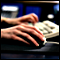Problems of Technical Analysis Revisited

At present, technical analysis along with the fundamental one is the most important method to analyze stock market. Being one of the predicting methods of stock market pricing dynamics, the technical analysis has a large amount of disadvantages that cast some doubt on its practical applicability.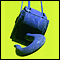Transferring an Indicator Code into an Expert Advisor Code. Indicator Structure

This article dwells on the ways of transferring an indicator code into an Expert Advisor Code and on writing Expert Advisors with no calling to custom indicators, and with the whole program code for the calculation of necessary indicator values inside the Expert Advisor. This article gives a general scheme of an indicator structure, emulation of indicator buffers in an Expert Advisor and substitution of the function IndicatorCounted(). The article is intended for readers, already having experience of programming in MQL4 language.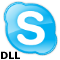Using Skype to Send Messages from an Expert Advisor

The article deals with the ways of how to send internal messages and SMSes from an Expert Advisor to mobile phones using Skype.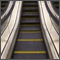Transferring an Indicator Code into an Expert Advisor Code. Conclusion

This is the final article, devoted to transferring an indicator code into an Expert Advisor code. Here the author transforms on a certain example a code of an Expert Advisor so, that this EA is presented in a single file without calling custom indicators.